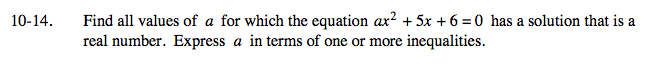### Home > A2C > Chapter 10 > Lesson 10.1.1 > Problem10-14

10-14.

Find all values of a for which the equation ax2 + 5x + 6 = 0 has a solution that is a real number. Express a in terms of one or more inequalities. Homework Help ✎Use the discriminant. Find the value of a that makes this quantity non-negative.

$a\le\frac{25}{24}$